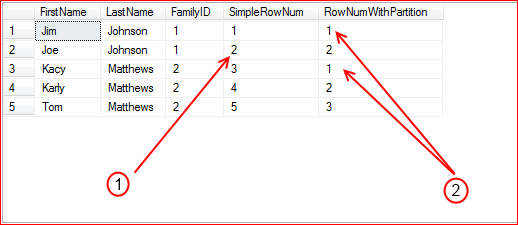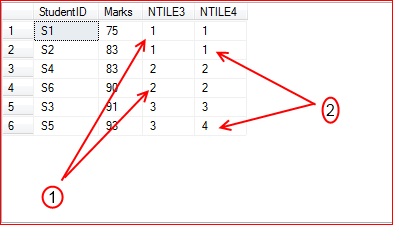# Ranking Funtions – Explained

Ranking function  help us assigning a rank value for each row in your result-set. They support the windowing the partition too. Books Online states that these functions are non-deterministic.

These are the ranking functions available in SQL Server.

RANK()
Returns the rank of each row within a result set. The rank of a row is one plus the number of ranks that come before the row in question. So RANK() may return values with gaps when there are ties. You could use the partition on OVER clause to have partitioning in the rank values.

DENSE_RANK()
Returns the rank of rows within the partition of a result set. The rank of a row is one plus the number of distinct ranks that come before the row in question. As the name suggests, DENSE_RANK returns the rank values without gaps and this is the difference between RANK() and DENSE_RANK().

ROW_NUMBER()
Returns the sequential number for each row within a result set. ROW_NUMBER always starts with one.

NTILE()
This distributes the result set into a specified number of groups. The groups are sequential numbers, starting at one. For each row, NTILE returns the number of the group to which the row belongs. The rows are evenly distributed into each group when the total rows in the result set is divisible by the number of groups specified. If this is not the case there size of group may vary.

Lets see how these ranking functions works

RANK() & DENSE_RANK()

Consider this example, Here we are trying to assign a rank to the salesperson depending on their sales in last 30 days

```SELECT S.SalesPersonID,
S.TerritoryID,
Count(S.SalesOrderID) TotalSales,
RANK() OVER (PARTITION BY S.TerritoryID
ORDER BY Count(s.salesOrderID) DESC) AS 'RANK',
DENSE_RANK() OVER (PARTITION BY S.TerritoryID
ORDER BY Count(s.salesOrderID) DESC) AS 'DENSE_RANK'
WHERE TerritoryID in (1,2) AND
OrderDate BETWEEN GetDate()-30 AND GetDate()
GROUP BY
S.SalesPersonID,S.TerritoryID```

The query gives the results as below and shows the main difference between the RANK and DENSE_RANK

1. RANK and DENSE_RANK assigned a ranking value with in the partition(TerritoryID)

2. RANK returned values that have gaps(in the case of ties)

3. DENSE_RANK produce a result without gapsROW_NUMBER()

In the query, row number  is assigned to each row, one with partition and other one without.

```SELECT
FirstName,
LastName,
FamilyID,
ROW_NUMBER() OVER(ORDER BY FirstName) AS SimpleRowNum,
ROW_NUMBER() OVER(Partition by FamilyID ORDER BY FirstName) AS RowNumWithPartition
FROM Person AS p
```

In the result, SimpleRowNum is a simple running number where as RowNumWithPartition is a running number with in a partition (FamilyID)However anyone wonder how you can assign the row numbers with out any specific ordering? There is no way you could avoid the ORDER BY clause, but you can specify query expression.

`ROW_NUMBER() OVER(Partition by FamilyID ORDER BY (SELECT NULL)) AS RowNumWithPartition`

NTILE()

In the example, we are trying to distribute the rows into 3 and 4 groups.

```SELECT *,
NTILE(3) OVER(ORDER BY Marks) NTILE3,
NTILE(4) OVER(ORDER BY Marks) NTILE4
FROM Student```

There are 6 rows, So NTILE3 creates groups having 2 rows each. However NTILE4 cannot distribute the rows equally among the groups, So it returns two group contain 2 rows in each at first and then another two groups having one row in each.You can download the entire source code used in the blog here

References
Ranking Functions (Transact-SQL)

## 2 thoughts on “Ranking Funtions – Explained”

1. Pingback: Stephen
2.best website solutions says:

very good and detailed post